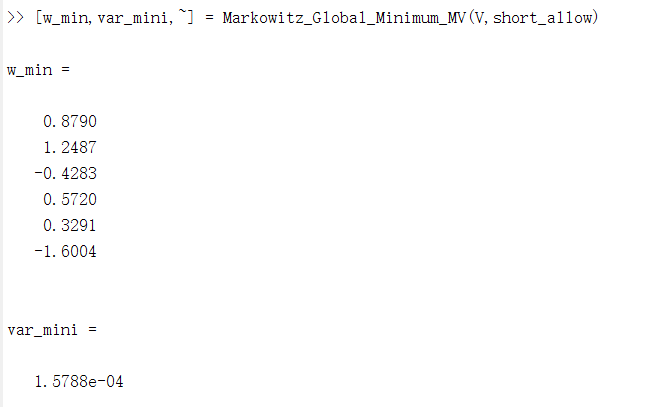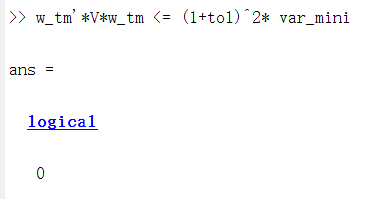# CVX 's solution doesn't satisfy constraint

I want to solve the following problem, the cvx gives me the solution, but this solution doesn’t satisfy the constraint ( w_tm’Vw_tm <= (1+tol)^2* var_mini)？

the input parameters are like this:

and tol= 0.1, short_allow=1

the main function is like this,

the called function gives me the minimize variance

I run this main function, the cvx gives me the solution like this,

in the main function, the called function give me the var_mini like this,but the optimal solution w_tm doesn’t satisfy the constraintI don’t know why ,really thanks for your help!

When optimality is claimed, the solvers called by CVX are only “guaranteed” to provide solutions which satisfy constraints to within a feasibility tolerance, which might typically be approximately 1e-8. If the constraint is violated by 1e-9, that is considered acceptable; but if that occurred in the constraint you claim is violated, it would produce logical 0 in your constraint satisfaction assessment. You should subtract one side of the inequality constraint from the other and look at the numerical value.

If you need a constraint to be strictly satisfied, even though the solver might violate it by a small amount, you need to include a buffer amount. Pick a small_positive _number, such as 1e-6; change
`LHS <= RHS`
into
`LHS + small_positive_number <= RHS`.
Then if CVX/solver claim the problem is solved to optimality, it is actually the case that `LHS <= RHS`.

Don’t use `quiet` until you are sure everything is working correctly. That way, you will see the solver and CVX output.